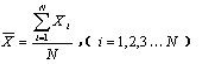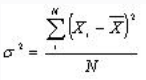• 详细介绍了matlab求方差和标准差的函数用法，包含示例代码
• % 用while方式输入n个数 当前数x,平方和sum2,和sum,最后平均数ave和标准差y clc;clear; n=input('请输入数据的总数'); N=n; disp(['接下来请按照提示依次输入',num2str(n),'个非负数。']); sum2=0 ;sum=0; count=1...
% 用while方式输入n个数  当前数x,平方和sum2,和sum,最后求平均数ave和标准差y
clc;clear;
n=input('请输入数据的总数');
N=n;
disp(['接下来请按照提示依次输入',num2str(n),'个非负数。']);
sum2=0 ;sum=0;
count=1;
x=0;
X=[];%用来存放x
while n>0
n=n-1;
disp(['现在开始请输入第',int2str(count),'个非负数']);
count=count+1;
x=input('');
sum2=sum2+x^2;
sum=sum+x;
disp(['您刚才输入的数是',int2str(x)]);
X=[X;x];
if x<0
break;
flag=1;
%flag原来是0,如果break后面的语句有执行，那么
%下面的提示信息会显示。
end
if flag==1
disp(['楼主如果看到这条信息,说明break之后的程序被执行']);
end
close all;
end
close all;
ave=mean(X);
y=std(X);
disp(['平均数是',num2str(ave),'标准差是',num2str(y)])
disp(['总数是',int2str(N),'总和是',int2str(sum),'平方和是',num2str(sum2)]);

展开全文• 1、均值 数学定义： Matlab函数：mean 如果X是一个矩阵，则其均值是一个向量组。mean(X,1)为列向量的均值，mean(X...按方差公式的不同，方差分为两种：biased（有效估计的方差unbiased（无偏估计的方差...
1、 均值

数学定义：Matlab函数：mean

如果X是一个矩阵，则其均值是一个向量组。mean(X,1)为列向量的均值，mean(X,2)为行向量的均值。若要求整个矩阵的均值，则为mean(mean(X))或者mean2(X)。

2、 方差

数学定义：均方差：

按求取方差公式的不同，方差分为两种：biased（有效估计的方差）和unbiased（无偏估计的方差）。当分析数据为样本数据（Sample Data）为无偏估计的方差，求方差除以n-1 ，分析数据为总体数据（The Population ）有效估计的方差，求方差除以n。无偏估计的方差可以修正样本的差异，更精确描述样本空间。因此matlab默认采用的是无偏估计的方差。

Matlab 函数：var

如果X是一个一维向量，var(data,1) 求总体方差，var(data,0) 求样本方差

如果X是一个矩阵，var没有求矩阵的方差功能，可使用std先求均方差，再平方得到方差。

3、 标准差

定义：

标准差是对均方差开根号。同理，标准差也分为两种，一种是总体数据（除n），一种为样本数据(除n-1)。

Matlab 函数：var

假设X是矩阵，那么

std1=std(X(:),1);%有效估计的方差（/n）

std2=std(X(:),0);%无偏估计的方差（/n-1）

std3=std2(X);%无偏估计的方差（/n-1）

展开全文• 首先，什么是方差和标准差？ 方差，是在概率论和统计方差衡量随机变量或一组数据时离散程度的度量，统计中的方差（样本方差）是每个样本值与全体样本值的平均数之差的平方值的平均数。在许多实际问题中，研究方差...
首先，什么是方差和标准差？

方差，是在概率论和统计方差衡量随机变量或一组数据时离散程度的度量，统计中的方差（样本方差）是每个样本值与全体样本值的平均数之差的平方值的平均数。在许多实际问题中，研究方差即偏离程度有着重要意义。
标准差，中文环境中又常称均方差，是离均差平方的算术平均数的平方根。标准差是方差的算术平方根。标准差能反映一个数据集的离散程度。平均数相同的两组数据，标准差未必相同。

1.方差

计算公式：在Matlab中使用方法是：

V=var(X,flag,dim)
参数解释如下：
X为矩阵或者向量;
flag为权值，当flag等于0时：前置因子是1/(n-1)，当flag等于0时：前置因子是1/(n)，默认是0；
dim为维数，当dim=1时，表示计算列，当dim=2时，表示计算行。

2.标准差

计算公式：在Matlab中使用方法是：

V=std(X,flag,dim)
参数解释如下：
X为矩阵或者向量;
flag为权值，当flag等于0时：前置因子是1/(n-1)，当flag等于0时：前置因子是1/(n)，默认是0；
dim为维数，当dim=1时，表示计算列，当dim=2时，表示计算行。

程序示例：

clc;clear;
matrix=[1, 2, 3; 4, 5, 6];

matrix_var_col=var(matrix); % 计算方差，权值为0，维度为1，也就是计算列方差
%matrix_var_col =
%    4.5000    4.5000    4.5000

matrix_var_row=var(matrix,0,2);% 计算方差，权值为0，维度为2，也就是计算行方差
%matrix_var_row =
%     1
%     1

matrix_std_col=std(matrix,1);% 计算标准差，权值为1，维度为1，也就是计算列标准差
%matrix_std_col =
%    1.5000    1.5000    1.5000

matrix_std_row=std(matrix,1,2);% 计算标准差，权值为1，维度为2，也就是计算行标准差
%matrix_std_row =
%    0.8165
%    0.8165
展开全文• MatLab在科学计算中，具有很强大的功能，常用的计算方法是计算一组数据的平均数，标准差方差。 1.计算一个数组的平均值 使用 mean() 函数 示例1： 输入：a = [ 1 2 3;4 5 6] 输入：mean(a) 输出：[ 2.5,3.5,...
文章目录概述1.计算一个数组的平均值2.计算一个数组的方差3.计算一个数组的标准差
概述
MatLab在科学计算中，具有很强大的功能，常用的计算方法是计算一组数据的平均数，标准差，方差。
1.计算一个数组的平均值
使用 mean() 函数
示例1：
输入：a = [ 1 2 3;4 5 6]
输入：mean(a)
输出：[ 2.5,3.5,4.5]
解析：默认是先求得每一列的的和，然后对每一个列求平均值
示例2：
输入：mean(mean(a))
解析：此时所输出的就是整个数组的平均值，需要加以注意
2.计算一个数组的方差
示例：
输入：var(a) % a 采用上面的示例
输出：[4.5,4.5,4.5]
解析：依然是求得每一列的方差
解决方法：将矩阵转换为列向量
输入：a = [1;2;3;4;5;6]
输入：var(a)
输出：[3.500]
3.计算一个数组的标准差
和计算方差一样，将数组转换为列向量，使用std(a)，进行求解
输入：a = [1;2;3;4;5;6]
输入：std(a)
输出：[1.8708]


展开全文• 本博文源于matlab基础，主要讲述常用统计量的计算包括均值、中位数、极差、方差和标准差数据分析 数据挖掘
• 方差:Y=var(X,0,1):矩阵X的列向量方差； Y=var(X,0,2):矩阵X的行向量方差 协方差（Covariance）在概率论统计学中用于衡量两个变量的总体误差。而方差是协方差的一种特殊情况，即当两个变量是相同的情况 .....
• 一、离差指单项数值平均值的差，正负可以作为方向。用来反映某一数值与平均值的偏离程度(距离)。二、标准差(sd)用来反映样本数据离散程度的指标。多用来反映样本是否“稳重”。...三、方差标准差平方后的值。一...
• 利用Matlab求矩阵的矩阵和标准差,简单易用，非常好的一段小程序。
• % 求标准差与相关系数 % 排序 % 矩阵的最大元素最小元素 % max():向量或矩阵的最大元素。 % min( ):向量或矩阵的最小元素。 % 1、当参数为向量时，函数有两种调用格式: % (1) y=max(X): 返回向量X的最大...
• mu*mu) skew = (m3 - 3*mu*m2 + 2*mu**3) / sigma**3 kurtosis = (m4 - 4*mu*m3 + 6*mu*mu*m2 - 4*mu**3*mu + mu**4) / sigma**4 - 3 print('手动计算均值、标准差、偏度、峰度：', mu, sigma, skew, kurtosis) # ...
• ## 复数求标准差

千次阅读 2012-12-07 15:26:41
今天自己写程序，复数集合的标准差，做了半天，终于和Matlab的std函数得出的值相一致。  其实很简单，就是将复数转为模值，把它看做是实数来做，  使用公式：D(X) = E(X^2) - E(X)^2，可得其方差。  其中： ...
• 一：matlab里面关于方差和协方差的几点需要注意的 求均值的函数：mean 求方差的函数：var 标准差： 方差： 求协方差的函数：cov 1 如果a,b是向量，则： cov(a,b)相当于是[D(a),cov...
• %SCR和方差的方法 clear,clc,close all imgName=input('请输入bmp文件名,要有单引号,要有后缀:'); img=imread(imgName); figure,imshow(img,[]) leftupPointRow=input('请输入目标的左上角的行数(第二坐标):'...
• 当分析数据为总体（Population）和样本（Sample）时方差和标准差的计算方式又有不同，简单可以记忆为样本数据（Sample Data）求方差除以n-1 ，总体数据（The Population ）求方差除以n ，公式如下： The Population...
• 标准差：std 方差：var 相关系数：corrcoef 排序：sort 四则运算 1.多项式的加减运算就是所对应的系数向量的加减运算，次数不同则用0补齐 2.用conv(p1,p2)计算多项式p1与p2的乘积。p1,p2为系数向量 3.计算多项式除法...
• 本节主要介绍MATLAB预定义函数，包括一般数学函数（指数，对数，根），近似函数，离散数学函数（因数函数...求和积，排序，方差和标准差），随机数产生函数（均匀分布的随机数，高斯分布随机数），与复数有关的函数。数学函数 数据分析
• %%学习目标 排序 最值 标准差 方差 %排序 clear all; x=[1 3 4;8 3 5;2 7 4] yl=sort(x) %按列从小到大排序 y2=sort(x,2) %按行从小到大排序 y3=sort(x,1,'descend') %按列从大到小排序 %最值 %%最大值...
• 样本变异系数：变异系数又称为离散系数，定义为标准差与平均值之比，样本变异系数即样本数据的标准差与其均值之比。样本k阶中心矩：在概率论中，矩是用来描述随机变量的某些特征的数字，即平均值；随机变量X的K阶...
• 八、数据分析付里叶变换（datafun）   1．基本运算 max 向量的最大分量 ...向量的最小分量 ...向量的平均值 ...向量的中值 ...标准差 var 方差Varifft filter 数据分析
• 1、基本统计量，均值，中位数，标准差方差 2、正太分布的概率密度图、概率、均值和方差，产生正太分布的随机数 x=-6:0.01:6; y=normpdf(x); z=normpdf(x,0,2); plot(x,y,x,z) 正态分布的均值假设检验 ...
• 3.2.16 var/std 求方差标准差/66 3.2.17 diag 生成对角矩阵/68 3.2.18 repmat 矩阵复制平铺/69 3.2.19 reshape 矩阵变维/70 3.2.20 inv/pinv 矩阵求逆/求伪逆/71 3.2.21 rank/det 求矩阵的秩/行列式/73 3.2.22 ...
• 8．4．4 方差和标准差 8．4．5 协方差与相关系数 8．5 统计作图 8．5．1 正整数频率表 8．5．2 累积分布函数图形 8．5．3 最小二乘拟合直线 8．5．4 绘制正态分布概率图形 8．5．5 样本数据的盒图 8．5．6 参考线...
• 8．4．4 方差和标准差 8．4．5 协方差与相关系数 8．5 统计作图 8．5．1 正整数频率表 8．5．2 累积分布函数图形 8．5．3 最小二乘拟合直线 8．5．4 绘制正态分布概率图形 8．5．5 样本数据的盒图 8．5．6 参考线...
• //SquarePoor:返回方差分析指标：回归平方，剩余平方，回归平方，剩余平方 //返回值：0求解成功，-1错误； int MultipleRegression(double *data, int rows, int cols, double *Answer, double *...c语言
• 即把一个向量的均值和标准差算出来，然后每一个分量都减去均值再除以标准差，得到的就是归一化之后的结果。 归一化之后的数据有正有负，服从均值为0方差为1的标准正态分布。 实验：归一化前后，数据分布不同 在...# matlab求方差和标准差matlab 订阅